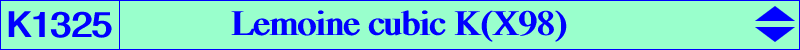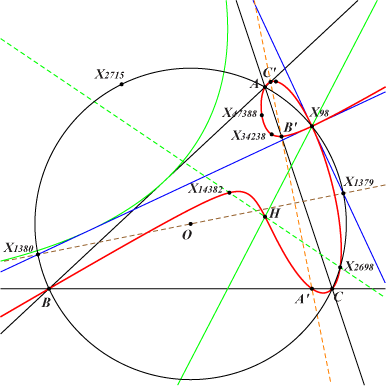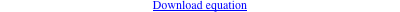too complicated to be written here. Click on the link to download a text file.X(4), X(98), X(2698), X(5967), X(9154), X(14382), X(34238), X(47388), X(53868), X(53869) A', B', C' : traces of the Simson line of X(98), passing through X(115), X(512) projections of X(98) on the altitudes infinite points of pK(X6, X147) Geometric properties :K1325 is the Lemoine generalized cubic K(X98) hence it is a nodal cubic with node X(98), the nodal tangents being perpendicular, passing through X(1379), X(1380) on the Brocard axis and on the circum-circle. See also Table 64. More generally, every Lemoine cubic K(P) with P on (O) is a cK. K1325 is the only Lemoine cubic which is a cK0, namely cK0(#X98, X2395). K1325 is the barycentric product X(98) × K185, and more generally, the barycentric multiple of the nine cubics mentioned in the page K979.   For any M on K1325, the cevian lines of M meet the corresponding lines passing through X(98) and A', B', C' at three collinear points on a line L(M). This line is tangent to the inscribed parabola (P) with focus X(2715), directrix the line X(4), X(98). (P) is tangent to the Brocard axis, to the nodal tangents and its dual conic is the circum-hyperbola with perspector X(2799), center X(35088), passing through X(i) for i = 2, 297, 325, 327, 850, 2857, etc. This is the isotomic transform of the line passing through X(i) for i = 2, 98, 110, 114, 125, 147, 182, 184, 287, 542, 1352, 1899, 1976, and many others.